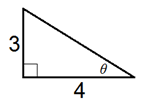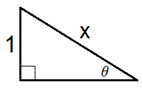# The Problems with Inverse Trig Problems

4 teachers like this lesson
Print Lesson

## Objective

SWBAT use inverse trig functions in a variety of contexts.

#### Big Idea

Highlight common hurdles of inverse trig functions to evoke deep thinking.

## Launch

8 minutes

At the start of class I will ask students how to find csc-1(5/2), a question from yesterday’s homework assignment. After a minute or so, I will ask students for some strategies to see how they plan to approach the problem. Some observations that may come up are listed below:

1. I don’t have an inverse cosecant button on my calculator.
2. Cosecant and sine are inverses, so I know it has something to do with that.
3. We are looking for an angle measure.
4. I know that this angle will not be a multiple of 30° or 45°.
5. If an angle has a cosecant of (5/2), then its sine ratio has to be (2/5).
6. The expression csc-1(5/2) is equivalent to sin-1(2/5), so we can just get the angle measure that way.

If students do not give these observations, ask them questions to get them thinking about these topics.This line of reasoning is very important for students to understand inverses, and it is also a good preface to the tasks we are working on today.

## Explore

15 minutes

One of my favorite educators, Dr. Rheta Rubenstein of University of Michigan-Dearborn, has a philosophy that if you cannot fix a misconception then you should feature it. By making a misconception a focal point, you can at least be very transparent about why it is confusing for students in hope of making the concept clear for the highest number of students. That is my intent today – to feature three concepts that are confusing for students in the hopes of finding clarity.

In this section of class I plan to give students the tasks and have them work in their table groups to solve. They may not be able to solve each task, but at least they will be primed and thinking about these concepts before we have our class discussion about them. Below are some notes about each one of the tasks.

Task #1: This will get students thinking about the range of outputs for the inverse trig functions. If students know that 300 degrees is not the solution, ask them why. Also, have them think about why a student might put that as an answer. You can also ask them why 300 degrees is not a solution when the cosine of 300 degrees is ½.

Task #2: Students may only give 60 degrees as a solution. See my video below for some explanation about this problem.

Unable to display content. Adobe Flash is required.

Task #3: If they are stuck, ask them to think about what the value in the parentheses represents. Since it is arctan3/4, it is an angle measure, so whatever that angle is, we want the sine of it. Some may use their calculator to find the angle whose tangent is ¾ and then will find the sine of that angle. If they do not round at all, they will get the correct answer. If they do round, the answer will not be correct. Challenge them to use a diagram to get the correct answer.

Task #4: This one is similar to 3, but there are variables instead of numbers. If students successfully found the answer to 3, ask them how these two questions are alike. Again, challenge them to draw a diagram to represent the situation. Also have them think about the fact that sin-1(1/x) is an angle, and we want the cosine of that angle.

## Share

20 minutes

After the exploration I have students present their work for the first two tasks. I make sure to hit on the six points raised in the Launch section. For Task #2, making sure that students understand that there are an infinite number of solutions to the equation. I conclude by asking students to identify specific angles that they can plug in to make the statement true. In this instance repetition is key to understanding (MP8).

For Tasks #3 and #4, I plan to challenge students to create a diagram to represent the situation. I want them to see that for #3, arctan ¾ is an angle measure, so all that we are looking for is the sine of the angle whose tangent is ¾. This can easily get lost in the notation, so make sure that students realize this. Setting up this diagram will definitely help – all we need to do is find the sine of θ.For Task #4, we can set up a similar diagram but we are just using variables. We need to find the cosine of θ. Students can then use Pythagorean Theorem to find the adjacent side, and then can set up their ratio.## Summarize

10 minutes

This assignment is a good summary of all of the work that we have done with inverse trig functions over the last few days. There is some conceptual work, but also the procedural practice for evaluating inverses.

Be careful of question 5d! When evaluating cot-1(-4) on the calculator, you will have to type in tan-1(1/-4) which will give you -14.04°, but that is not in the range of the inverse cotangent function, so you will have to add 180° to get an acceptable answer.